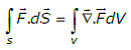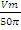# given a vector field the divergence theorem states that

1.

2.

3.

4.

4Explanation :
No Explanation available for this question

# if a 400V,50 Hz star connected 3 phase squirrel cage induction motor is operated from a 400 V,75 Hz supply the torque that the motor can now provide while drawing rated current from the supply

1.  reduces

2.  increases

3.  remains the same

4.  increase or reduces depending upon the rotor resistance

4

reduces

Explanation :
No Explanation available for this question

# A dc series motor fed from rated supply voltage is overload and its magnetic circuit is saturated.the torque-speed characteristic of this motor will be approximately represented by which curve of below fig

1.  curve A

2.  curve B

3.  curve C

4.  curve D

4

curve B

Explanation :
No Explanation available for this question

# A 1 kVA,230V/100V single phase 50 Hz transformer having negligible winding resistance and leakage inductance is operating under saturation while 250V,50V Hz sinusoidal supply is connected to the high voltage winding.A resistance load is connected to the low voltage winding which draws rated current.which one of the following quantities will not be sinusoidal

1.  voltage induced across the low voltage winding

2.  core flux

4.  current drawn from the source

4

current drawn from the source

Explanation :
No Explanation available for this question

# A 400 V/200V,50Hz three winding transformer is connected as shown the figure,the reading of the voltmeter V will be

1.  0V

2.  400 V

3.  600 V

4.  800 V

4

0V

Explanation :
No Explanation available for this question

# 10the frequency of the clock signal applied to the rising edge triggered D flip-flop shown in figure is 10kHz.the frequency of the signal available at Q is

1.  10 kHz

2.  2.5 kHz

3.  20 kHz

4.  5 kHz

4

5 kHz

Explanation :
No Explanation available for this question

# The forward resistance of the diode shown in figure is 5 and the remaining parameters are same as those of an ideal diode,the dc component of the source current is

1.

2.

3.

4.

4Explanation :
No Explanation available for this question

# the cut-in voltage of both zener diode D2 and diode D shown in figure is 0.7V,while break down voltage of D2 IS 3.3 V and reverse breakdown voltage of D is 50 V the other parameters can be assumed to be the same as those of an ideal diode.the values of the peak output voltage (Vo) is

1.  3.3 V in the positive half cycle and 1.4 in the negative half cycle

2.  4 V in the positive half cycle and 5V in the negative half cycle

3.  3.3 V in both positive and negative half cycles

4.  4V in both positive and negative half cycles

4

4 V in the positive half cycle and 5V in the negative half cycle

Explanation :
No Explanation available for this question

# the line-to-line input voltage to the 3 phase 50 Hz ac circuit shown in the figure is 100 V rms. Assuming that the phase sequence is RYB, the wattmeters would read.

1.  W1=886 W and W2=886 W

2.  W1=500 W and W2=500 W

3.  W1=0 W and W2=1000 W

4.  W1=250 W and W2=750 W

4

W1=0 W and W2=1000 W

Explanation :
No Explanation available for this question

1.  E000-EFFF

2.  000E-FFFE

3.  1000-FFFF

4.  0001-FFF1

4